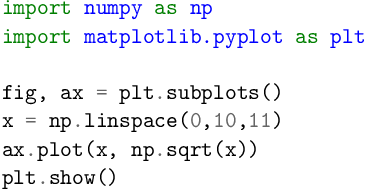# The minted Package

Documentation code The minted package creates nicely formatted and colorized code listings. It requires that you run pdflatex with the flag -shell-escape. \usepackage{minted}

To include a python code example, use the following:

\begin{minted}{python}
import numpy as np
import matplotlib.pyplot as plt

fig, ax = plt.subplots()
x = np.linspace(0,10,11)
ax.plot(x, np.sqrt(x))
plt.show()
\end{minted}